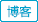#1. 云栖社区>
2. 全部标签>
3. #DataFrame####Python常见数据框操作①

import numpy as np import pandas as pd from pandas import Sereis, DataFrame ser = Series(np.

###pandas VS baseR

import numpy as np import pandas as pd 创建DataFrame In : df = pd.DataFrame({'col_a': np.

###Python数据分析之读取文件

Python的数据分析，大部分的教程都是想讲numpy，再讲Dataframe，再讲读取文件。但我看书的时候，前面二章看的实在头晕，所以，我们还是通过读取文件来开始我们的Python数据分析吧。

25
GO